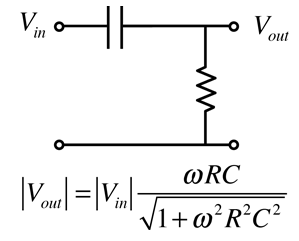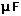RC High Pass Filter

Since capacitive reactance decreases with frequency, the RC circuit shown discriminates against low frequencies. The circuit is an AC voltage divider with an output which falls off at low frequencies at the rate of 6 dB per octave.CalculationMore detail

It is typical to plot filter response curves in decibels, which are defined in terms of power or intensity ratios. They are defined as 10 times the logarithm to the base 10 of the power ratio. The electric power dissipated in a resistor is proportional to the voltage squared, so if you are using a voltage ratio instead, the number of decibels is 20 log10(Vout/Vin).

 Filter Circuits
Index

Capacitance concepts

Inductance concepts

 HyperPhysics*****Electricity and magnetism R Nave
Go Back

RC High Pass Filter DetailsRC high pass filter Calculation
 Filter Circuits
Index

Capacitance concepts

Inductance concepts

 HyperPhysics*****Electricity and magnetism R Nave
Go Back

RC High Pass Filter Calculation

Since capacitive reactance decreases with frequency, the RC circuit shown discriminates against low frequencies. The circuit is an AC voltage divider with an output which falls off at low frequencies at the rate of 6 dB per octave.More detail

One approach to the design of a high-pass filter is to establish a frequency "breakpoint" or "knee" by choice of the resistance and capacitance. The design calculation works by clicking on the desired quantity in the expression. Enter the necessary data and then click on the quantity you wish to calculate. Default values will be entered for unspecified quantities, but all quantities may be changed. After the filter has been designed, the amount of attenuation in decibels at any frequency can be evaluated by the separate calculation below. Once you get well below the breakpoint frequency, the amount of attenuation will increase by about 6dB per octave, which you can confirm with that exploratory calculation.

Breakpoint = 1/(ResistancexCapacitance)

 For R = x10^ Ω = kΩ = MΩ
 and C = x10^ F == pF
 the breakpoint angular frequency ω = x10^ rad/s
and frequency = x10^ Hz = kHz = MHz

and the capacitive reactance at the breakpoint is

 XC = x10^ ohms = kohms = Megohms

Evaluation of filter action:
 If the input angular frequency ωin = x10^ rad/s
 the output ratio Vout/Vin = x10^ = dB.

 Filter Circuits
Index

Capacitance concepts

Inductance concepts

 HyperPhysics*****Electricity and magnetism R Nave
Go Back